# Understanding Gunn-Peterson through the Reionization epoch

• I
Into an extra-galactic physics course, I don't understand well the "Gunn - Peterson" effect on the spectrum of high redshift quasars (z greater than 3 or 4, up to the current limit of z = 6-7 I think)

The observed fact is that there is an almost total absorption for the photons of the blue part of the Lyman-alpha line (1216 Angstrom). This is illustrated on the following 2 spectra: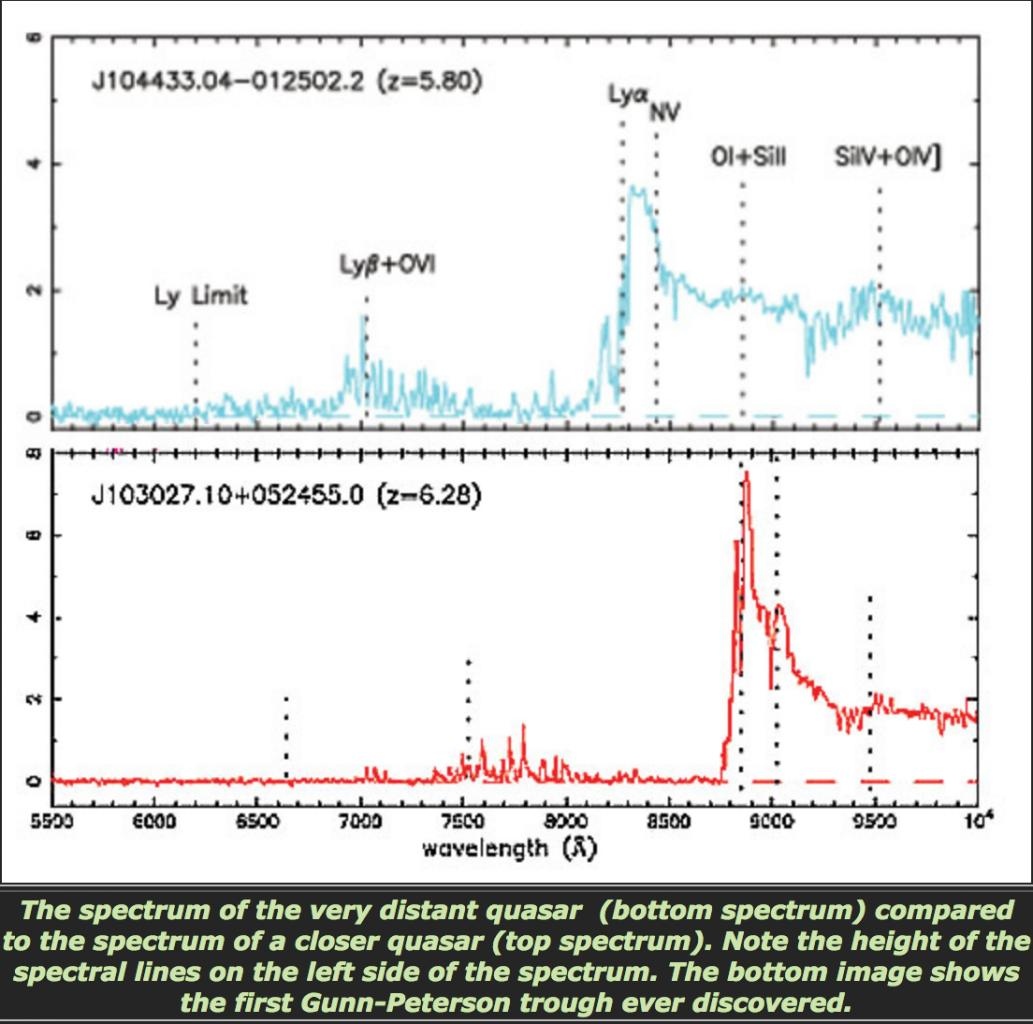You can see on the 2 spectra that for wavelengths lower than the Lyman-alpha emission line (1216 Angstrom) :

1) is the near surrounding of quasar is a thin or thick medium : since the Lyman-alpha seems to be an emission line (1216 Angstrom), I would say a first sight, that surrounding medium of quasar is thin.

2) Does the large "plateau" reflect the absorption of all photons in this range of lengths. 'wave ?

There are a few emission lines but a low height.

I have seen from my research that this absorption up to z = 6 would translate the end of the reionization epoch, that is to say the time when the first stars were formed and which ionized again the hydrogen atoms (after the first ionization that took place before the recombination):

3) I don't understand this relation between "end of the reionization" and all the blue part, before the line Lyman-alpha of the observed spectrum, which is absorbed.

The video below shows the evolution of the spectrum during the course of a flow of photons from the far quasar which is slowly redshifting while showing the absorption lines of the Lyman forest :

4) We don't see Lyman forest on the 2 spectrum at the begining of my post, do we ?

I understand that the hydrogen placed near the Lyman-alpha line is absorbed because the hydrogen gas close to the quasar absorbs the photons of the quasar, but :

5) What about the photons of shorter wavelengths? why do not they appear in the spectrum observed?

If, according to the line of sight, there are H+ ionized hydrogen atoms, then the quasar photons should not be absorbed, right? it would mean that there is little H+ on the line of sight, and so we could make the connection between the low density of H+ and the end of the reionization?

any help in understanding this effect is welcome.

sorry, the image was not displaying, here the original post with valid image :

Into an extra-galactic physics course, I don't understand well the "Gunn - Peterson" effect on the spectrum of high redshift quasars (z greater than 3 or 4, up to the current limit of z = 6-7 I think)

The observed fact is that there is an almost total absorption for the photons of the blue part of the Lyman-alpha line (1216 Angstrom). This is illustrated on the following 2 spectra: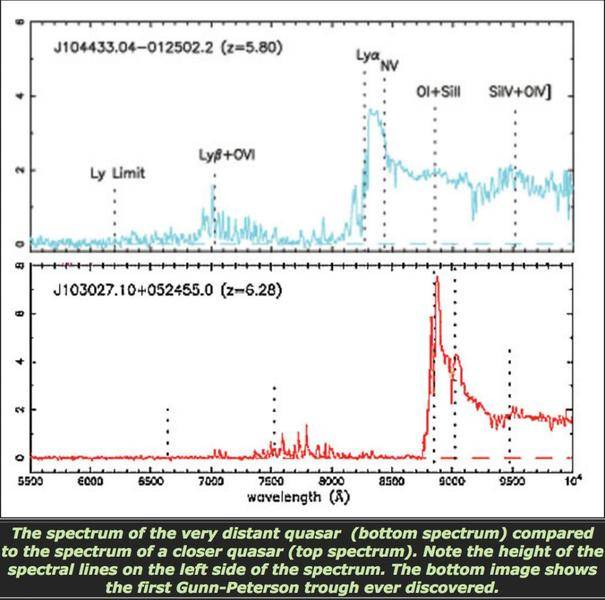You can see on the 2 spectra that for wavelengths lower than the Lyman-alpha emission line (1216 Angstrom) :

1) is the near surrounding of quasar is a thin or thick medium : since the Lyman-alpha seems to be an emission line (1216 Angstrom), I would say a first sight, that surrounding medium of quasar is thin.

2) Does the large "plateau" reflect the absorption of all photons in this range of lengths. 'wave ?

There are a few emission lines but a low height.

I have seen from my research that this absorption up to z = 6 would translate the end of the reionization epoch, that is to say the time when the first stars were formed and which ionized again the hydrogen atoms (after the first ionization that took place before the recombination):

3) I don't understand this relation between "end of the reionization" and all the blue part, before the line Lyman-alpha of the observed spectrum, which is absorbed.

The video below shows the evolution of the spectrum during the course of a flow of photons from the far quasar which is slowly redshifting while showing the absorption lines of the Lyman forest :

4) We don't see Lyman forest on the 2 spectrum at the begining of my post, do we ?

I understand that the hydrogen placed near the Lyman-alpha line is absorbed because the hydrogen gas close to the quasar absorbs the photons of the quasar, but :

5) What about the photons of shorter wavelengths? why do not they appear in the spectrum observed?

If, according to the line of sight, there are H+ ionized hydrogen atoms, then the quasar photons should not be absorbed, right? it would mean that there is little H+ on the line of sight, and so we could make the connection between the low density of H+ and the end of the reionization?

any help in understanding this effect is welcome.

#### Attachments

please, even a succint explanation to my questions would be enough

Bandersnatch
1) is the near surrounding of quasar is a thin or thick medium : since the Lyman-alpha seems to be an emission line (1216 Angstrom), I would say a first sight, that surrounding medium of quasar is thin.
The Lyman-alpha is both an emission and absorption feature. The largest peak shown in the continuous quasar spectrum is the emission line, while the forest consists of multiple Lyman-alpha absorption lines. There are good reasons to think that the surroundings are thin, so that the absorption is not from gas in the immediate vicinity of the observed quasar but from filaments of neutral hydrogen further along the path of light.

2) Does the large "plateau" reflect the absorption of all photons in this range of lengths. 'wave ?

There are a few emission lines but a low height.
The plateau is the G-P trough. It shows complete absorption in one specific wavelength (Lyman-alpha), corresponding to there being enough neutral gas density to block all light at this particular wavelength. Without expansion redshifting the entire spectrum, this would result in complete removal of light at 1216 Angstrom only. But since the spectrum is being redshifted as it travels, the part that used to be shorter is now in the absorption range, and is again fully absorbed.
If the density of neutral hydrogen is low, the absorption will not be full, and some light of the original spectrum will get through. The small peaks seen in the through (before the L-beta marker) are not emission lines, but what remains after incomplete absorption. The only emission peak shown to the left of L-alpha is the L-beta (which is also attenuated by analogous absorption).
As long as the remains of the original spectrum are still present, the absorption lines cut into it are the 'forest'. When the absorption lines become so strong and dense that the entire part of the original spectrum disappears - that's no longer a forest, but the G-P trough (the forest is so dense that you can't see anything through the trees).

I have seen from my research that this absorption up to z = 6 would translate the end of the reionization epoch, that is to say the time when the first stars were formed and which ionized again the hydrogen atoms (after the first ionization that took place before the recombination):

3) I don't understand this relation between "end of the reionization" and all the blue part, before the line Lyman-alpha of the observed spectrum, which is absorbed.
At the end of reionsation, the density of neutral hydrogen along the path of light would have started to be low enough for the Lyman-alpha absorptions to become rare enough to no longer completely remove the L-alpha wavelength - this means that once the reionisation was nearing completion, we should start seeing a forest instead of a trough, as some light in 1216A should start getting through.
So, if we look at progressively higher z quasars, and we see the lowest z where the through first appears ('first' means last, as we're looking back in time with each increment of z), it should indicate that this marks the moment in time when the intervening gas was last opaque at the L-alpha wavelength.

4) We don't see Lyman forest on the 2 spectrum at the begining of my post, do we ?
That's right. The second spectrum shows the G-P trough. That is, the 'forest' of absorption lines is so thick, that it completely erases that part of the original spectrum.

I understand that the hydrogen placed near the Lyman-alpha line is absorbed because the hydrogen gas close to the quasar absorbs the photons of the quasar, but :

5) What about the photons of shorter wavelengths? why do not they appear in the spectrum observed?

If, according to the line of sight, there are H+ ionized hydrogen atoms, then the quasar photons should not be absorbed, right? it would mean that there is little H+ on the line of sight, and so we could make the connection between the low density of H+ and the end of the reionization?
(I'm slightly repeating myself here)
Take a look at the continuous spectrum, shown in the video as the initial contour, with some emission peaks visible - the most prominent one being Lyman-alpha. The first cut to the left of the L-alpha peak is when the spectrum encounters some nearby neutral hydrogen on its way, and is attenuated. In non-expanding universe, this absorption line would be exactly at 1216A, and that's that. But the universe is expanding as the light travels, so the entire spectrum becomes redshifted. Now, the part of the spectrum previously in the range 1215A has become shifted to 1216A, so it's again subjected to L-alpha absorption. If there's a lot of neutral hydrogen encountered by the beam while this bit of the spectrum is in 1216A, this wavelength will once again become completely removed. Then the light travels some more, and what was originally emitted as 1214A light is now 1216A, so again L-alpha absorption takes place, and again is as efficient as how much gas is there in the way.

Also, have a look at Astrobites' articles on this subject:
https://astrobites.org/2013/07/14/astrophysical-classics-neutral-hydrogen-in-the-universe-part-1/
Part 2 is on the G-P trough. The numerous animations and graphs help a lot.

@Bandersnatch

Just a little precision, you can find below an image (sorry notations are in french but words are "similar") highlighting the fact that opacity (that we can assimilate to optical depth, can't we ?) is maximum when the gaz (in my case) is partially ionized :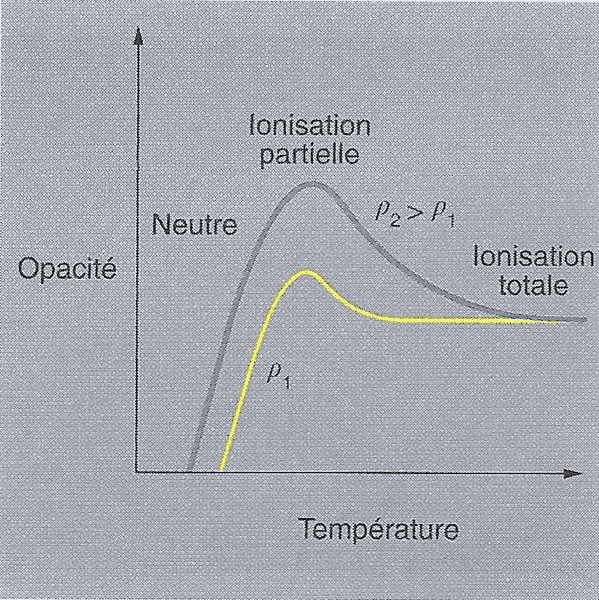Question 1) Concerning the Gunn-Peterson trough, is this law applied to explain the total absorption of Lyman-alpha into Quasar (z>6) spectrum ?

Here is how I am trying to understand :

I) Before recombination, the universe is opaque to light, ionization is total

II) After recombination, universe is transparent and fully neutral hydrogen

III) After Dark ages, locally temperature is increasing and so some gaz of hydrogen becomes partially ionized

Question 2) But with quasar very further (z>6), the Lyman forest is not present since opacity is maximal (from the opacity on figure above) : so the Lymann forest will be totaly absorbed by this high opacity : this would be the proof of Gunn-Peterson trough : is this correct ?

Question 3) On astrobites, there is the following figure :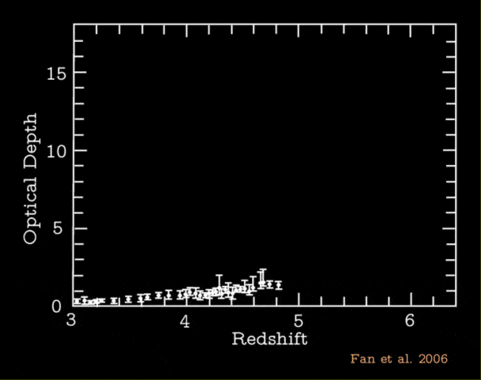Question 3) How optical predicted depth is computed (i.e the solid yellow line) ? Is it simply a Beer-Lambert law as a function of redshift z ?

Question 4) The optical depth experimental data are computed by depth and width of absorption rays "Lyman-alpha", "Lyman-beta" etc ..., is it right ?

Question 5) Does it mean that during the travelling of photons, the most time they travell, the most the intensity of flux will be small (since there will be a lot of absorption on the line of sight) ?

Question 6) Concerning the following figure of astrobites :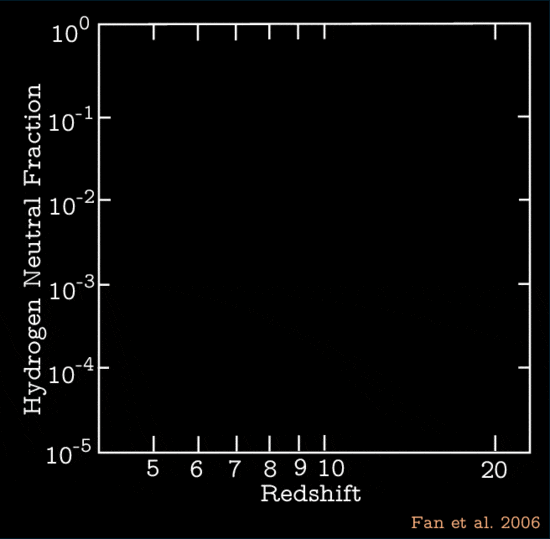We can see that fraction of neutral hydrogen in intergalactic medium is dropping around z=6 (approximately fraction = 10^-4) :

at z=0 (I mean today), is this always the case (the same value 10^-4 ?) ?

Sorry for my long post but the subject is very interesting and I would really like to understand all this history about reionization.

Any clarification is welcome, regards

#### Attachments

my last post is not only addressed to @Bandersnatch, any answer would be welcome.

something is bad questionned in my last post ? this would be fine to try to make me grasp these subtilities

Bandersnatch
Your questions are fine, if sometimes a bit hard to read. The reason there was no follow up from me, is that I'm not comfortable speaking about what I don't understand well enough. But it would appear that the PF members more knowledgeable in the subject are currently busy elsewhere, so, for what it's worth:

Re.: 1&2, and I think 5 too:
I don't think your first graph has much to do with the forest. The point about the absorption lines is that these are in specific wavelengths. You can expect that a photon emitted at recombination has a chance of not getting to your telescope because it may hit some intervening plasma and get scattered. That's what the regular optical depth is about - the more gas there is on the photon's path, i.e., the longer it had to travel, the higher the chance of attenuation. But with the forest, the attenuation is specific to those particular few absorption lines.
So, if there were no absorption lines, you'd have just the solid line shown in the second graph (Q3), indicating that there is some gas in whatever state that attenuates all wavelengths of light as it travels, roughly equally, with higher z sources being more attenuated just because there was more gas in the way (i.e., the optical depth increases).
With absorption, the optical depth increases in the GP wavelength range above that of the rest of the spectrum, because of the neutral hydrogen removing those particular wavelengths.

Re.: 3&4
Have a look at the Fan et al. study from which the Astrobites graphs are sourced. It's available on arxiv. If I understand it correctly, the curve is a power law best-fit to the lower-z data. They provide the equations in the paper under the relevant graphs.

As for 6 - I think it follows the curve as shown, monotonically decreasing, and approaching some value between 10E-4 and 10E-5. It's not like the remaining neutral gas can not become ionised over time.

Again, these are mostly just informed guesses. If you read the Fan et al. paper, maybe follow some of its references, you'll know more than I do.

The time of reionization seems to end at z = 5-6 (from the quasars and the Gunn-Peterson plateau for quasars with z> 6)

From my previous image (below) representing the fraction of neutral hydrogen according to the redshift, we see the very marked increase of the Hydrogen fraction for z> 5.I just wanted to know what is the shape of this curve for a redshift between z = 0 (today) and z = 5: a priori, the Hydrogen fraction should increase with time (for z decreasing) until to obtain a majority fraction close to about 10% of critical density ( I am not sure that today, the fraction is near to 10% on large scale) for today (z = 0)

But I would like to know what is the current value of this fraction of hydrogen, it would allow me to have the evolution of this value between z = 0 and z = 5.

If the curve (from z = 0 to z = 10) looks like, for example, to ##fraction = (z-z_{0})^2+b##, then it would allow us to deduce the epoch of the end of reionization located in the hollow of this type of curve (end of the reionization would be equal to ##z=z_{0}## with the minimal fraction of neutral hydrogen equal to ##fraction =b##)

I have a first element of answer on the following graph (from Fan et al. paper) https://arxiv.org/pdf/1105.2294.pdf) :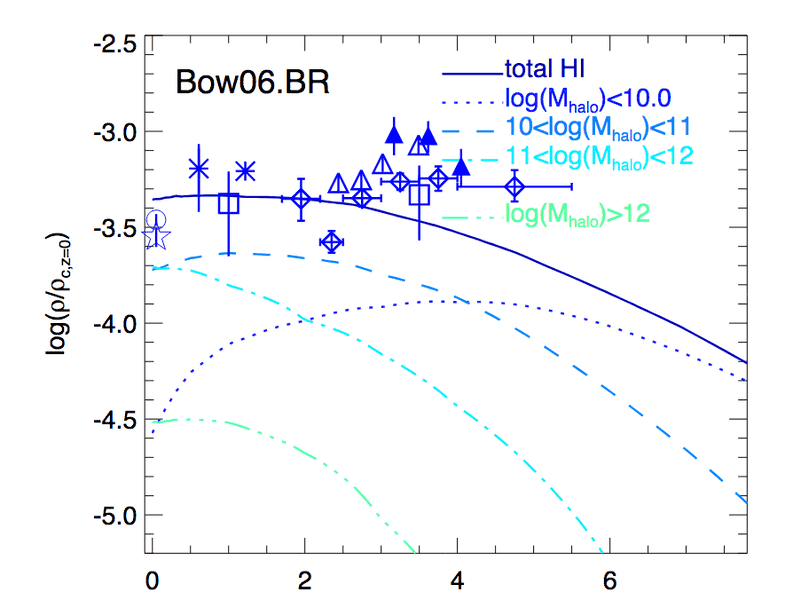As you can see, when redshift decreases, then the fraction is increasing, after the decreasing up to the end of reionization (proved by Gunn-Peterson effect on the black graph).

I have a last question : why the fraction of neutral hydrogne is equal to ##10^{-3.5}## at ##z = 5## (just after reionization) : is it du to the fact that one takes the ratio ##fraction=\dfrac{\rho}{\rho_c^0}## ?

Why don't we have today , on this graph above, ##fraction_{today} = 0.05## as baryonic content in universe is equal to 5% of the critical density ?
I don't understand this value around ##10^{-3.5}## for current fraction of hydrogen (in solid blue at z=0)

Regards

#### Attachments

Last edited:
To complete my questions, I wonder if the graph below is realistic (Neutral hydrogen fraction versus redshift) :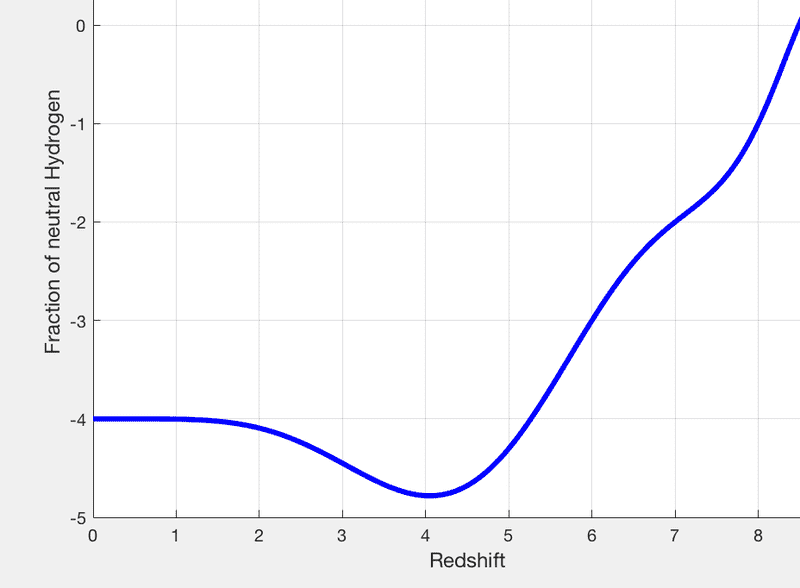this way, if we consider a redshift greater than 5-6, there are enough neutral hydrogen to get a a total absorption of Lyman-Forest, and so get a Gunn-Peterson through.

For redshift lower than 5-6, there are not enough neutral hydrogen to have a full absorption and so it always appears a Lyman forest in this case (like it is the case for quasar with redshift z < 5).

The values in this figure may be wrong, it is the shape (correct or not) which is important for me.

Any remark is welcome.

#### Attachments

kimbyd
Gold Member
I just wanted to know what is the shape of this curve for a redshift between z = 0 (today) and z = 5: a priori, the Hydrogen fraction should increase with time (for z decreasing) until to obtain a majority fraction close to about 10% of critical density ( I am not sure that today, the fraction is near to 10% on large scale) for today (z = 0)
The neutral hydrogen fraction should be pretty close to constant after z=5 or so. The physical process which drove reionization was that as stars started turning on, there were enough high-energy photons moving around to ionize the very low-density intergalactic medium. As there are still lots of bright stars around, low-density hydrogen is basically always ionized now.

If you're curious about ionization in the nearby universe, this paper may be useful:
https://www.aanda.org/articles/aa/full_html/2009/34/aa11811-09/aa11811-09.html

The basic conclusion is that in the late universe the primary thing determining ionization is the local density. As hydrogen clouds get more dense, the outer parts of the cloud shield the inner parts from the higher-energy photons, allowing neutral hydrogen to exist.

Note that these clouds are very low-density structures that exist between galaxies, and are generally much larger than individual galaxies.

You're not likely to find much info about the average neutral hydrogen fraction, because it's no longer a physically-meaningful number. Ionization in today's universe is all about local structure.

•Astronuc and Bandersnatch